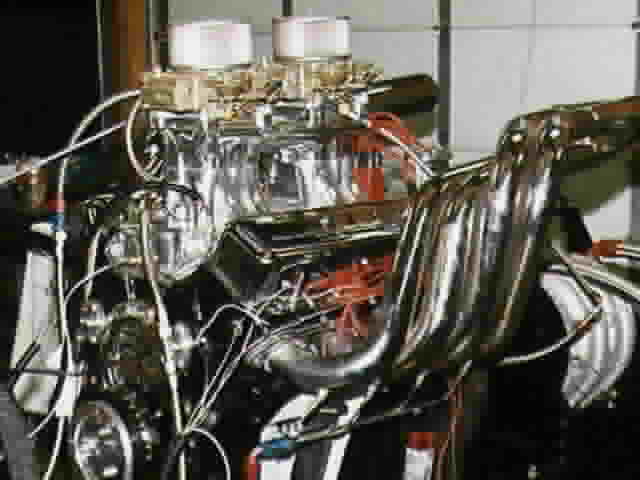Torque vs Horsepower

The word horsepower was introduced by James Watt, the inventor of the steam engine in about 1775. Watt learned that "a strong horse could lift 150 pounds a height of 220 feet in 1 minute." One horsepower is also commonly expressed as 550 pounds one foot in one second or 33,000 pounds one foot in one minute. These are just different ways of saying the same thing. Notice these definitions includes force (pounds), distance (feet), and time, (minute, second). A horse could hold weight in a static position but this would not be considered horsepower, it would be similar to what we call torque. Adding time and distance to a static force (or to torque) results in horsepower. RPM, revolutions (distance) per minute (time), is today's equivalent of time and distance. Back to horses, imagine a horse raising coal out of a coal mine. A horse exerting one horsepower could raise 550 pounds of coal one foot every second.

Here is an example of another way horsepower could be directly measured. Say you have a horse hitched to a plow. In the hitch is a spring scale (like a fish scale). The horse pulls the plow one foot every second and you see 550 pounds on the scale. That horse would be generating one horsepower.

We see horsepower can be directly measured. However there is a problem directly measuring horsepower of modern day internal combustion engines because they produce rotary motion not linear motion, and unless the engine is geared down, the speed at which they do work (time and distance or RPM) is too great for practical direct measurement of horsepower. It seems logical then that the solution was to directly measure torque (rotational force eventually expressed in pounds at one foot radius) and RPM (time and distance, i.e. distance in circumference at the one foot radius) and from these calculate horsepower. Torque and RPM are easily measured directly. Early dynamometers used a brake device to load the engine. A torque arm was attached to this brake's stator. The brake's rotor was coupled to the engine's crankshaft. A spring scale or other measuring device connected the torque arm to the stationary fixture holding the engine and brake. During a test the brake's application loaded the engine. Torque and engine rpm were observed and recorded. Click here for a description of how this happens on our dyno.

On modern day dynamometers horsepower is a calculated value. It's important to remember the dyno measures torque and rpm and then from these calculates horsepower. On the dyno it takes more water flow to the water brake to increase the load on the engine being tested. As the test engine's torque rises more water flow is needed. As the test engine's torque drops less water flow is needed. The dyno's water brake does not respond to Horsepower. Major adjustments to water flow are needed as an engine crosses its torque peak but none are needed as it crosses its horsepower peak. In other words the water flow to the brake during a dyno test follows the engines torque curve and not its horsepower curve. Torque is what twists the tire, prop, or pump. Horsepower helps us understand an amount or quantity of torque. (Torque + time and distance)

Now if we are measuring torque and RPM how can we calculate horsepower? Where does the equation HP=TORQUE X RPM / 5252 come from? We will use Watts observation of one horsepower as 150 pounds, 220 feet in one minute. First we need express 150 pounds of force as foot pounds torque.

(The * symbol means multiply in the explanations below.)

• Pretend the force of 150 pounds is "applied" tangentially to a one foot radius circle. This would be 150 foot pounds torque.

Next we need to express 220 feet in one minute as RPM.

• The circumference of a one foot radius circle is 6.283186 feet. ft. (Pi * diameter; 3.141593 * 2 feet)
• The distance of 220 feet, divided by 6.283185 feet, gives us a RPM of 35.014.

We are then talking about 150 pounds of force (150 foot pounds torque), 35 RPM, and one horsepower.

Constant (X) = 150 ft.lbs. * 35.014 RPM / 1hp

35.014 * 150 / 1 = 5252.1

5252 is the constant.

So then hp = torque * RPM / 5252

Here is another way; Remember we know 150 foot pounds and 35.014 RPM = one horsepower

1hp is to 150 ft.lbs. * 35.014 RPM as X hp is to observed ft.lbs.torque * observed RPM

Example; We dyno test and observe 400 ft.lbs. torque at 5000 RPM

1 hp is to 150 ft.lbs. * 35.014 RPM as X hp is to 400 ft.lbs. * 5000 RPM

When we cross multiply X hp * (150 ft.lbs. * 35.014 RPM) = 1hp * (400 ft.lbs. * 5000 RPM)

X hp * (5252 ft.lbs. RPM) = 1 hp * (2,000,000 ft.lbs. RPM)

Divide both sides by 5252 ft.lbs. RPM

X hp = 1 hp * 380.80

X hp = 380.80 hp

Horsepower = torque X rpm / 5252

Here's an interesting bit of trivia; below 5252 rpm any engine's torque number will always be higher than its horsepower number, and above 5252 rpm any engine's horsepower number will always be higher than its torque number. At 5252 rpm the horsepower and torque numbers will be exactly the same.

I've been told it also involves something called "radians". (See a definition of radians in the comments link below) I am not an engineer so my explanation may not be technically correct with out using "radians".

Comments from others on this subject.

If you have any questions please e-mail me the current address is on the RevSearch Home Page.

Check out the RevSearch Engine Dynamometer home page for more information on high performance engine building and dyno testing.